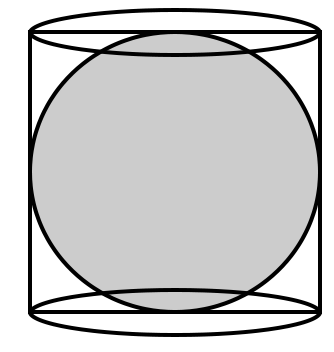# The ratio of the surface area of a sphere and the curved surface area of the cylinder circumscribing the sphere is :

A. 1 : 1

B. 1 : 2

C. 2 : 1

D. 2 : 3

### Solution(By Examveda Team)Let the radius of the sphere be r
Then, radius of the cylinder = r
Height of the cylinder = 2r
Surface area of sphere = $$4\pi {{\text{r}}^2}$$
Surface area of the cylinder = $$2\pi {\text{r}}(2r) = 4\pi {{\text{r}}^2}$$
∴ Required ratio :
= $$4\pi {{\text{r}}^2}$$ : $$4\pi {{\text{r}}^2}$$
= 1 : 1
Related Questions on Volume and Surface Area